• 公路运量主要包括公路客运量和公路货运量两个方面。根据研究，某地区的公路运量主要...请利用BP神经网络预测该地区2010年和2011年的公路客运量和公路货运量。 表5-11 运力数据表 年份 人数 机动车数量 公路面积 公里客

公路运量主要包括公路客运量和公路货运量两个方面。根据研究，某地区的公路运量主要与该地区的人数、机动车数量和公路面积有关，表5-11给出了某个地区20年的公路运量相关数据。根据相关部门数据，该地区2010年和2011年的人数分别为73.39万和75.55万，机动车数量分别为3.9635万辆和4.0975万辆，公路面积分别为0.9880万平方千米和1.0268万平方千米。请利用BP神经网络预测该地区2010年和2011年的公路客运量和公路货运量。
表5-11 运力数据表
年份 人数 机动车数量 公路面积 公里客运量 公里货运量
1990 20.55 0.6 0.09 5126 1237
1991 22.44 0.75 0.11 6217 1379
1992 25.37 0.85 0.11 7730 1385
1993 27.13 0.9 0.14 9145 1399
1994 29.45 1.05 0.2 10460 1663
1995 30.1 1.35 0.23 11387 1714
1996 30.96 1.45 0.23 12353 1834
1997 34.06 1.6 0.32 15750 4322
1998 36.42 1.7 0.32 18304 8132
1999 38.09 1.85 0.34 19836 8936
2000 39.13 2.15 0.36 21024 11099
2001 39.99 2.2 0.36 19490 11203
2002 41.93 2.25 0.38 20433 10524
2003 44.59 2.35 0.49 22598 11115
2004 47.3 2.5 0.56 25107 13320
2005 52.89 2.6 0.59 33442 16762
2006 55.73 2.7 0.59 36836 18673
2007 56.76 2.85 0.67 40548 20724
2008 59.17 2.95 0.69 42927 20803
2009 60.63 3.1 0.79 43462 21804
注：数据取自《Matlab在数学建模中的应用（第2版）》，卓金武，第134页。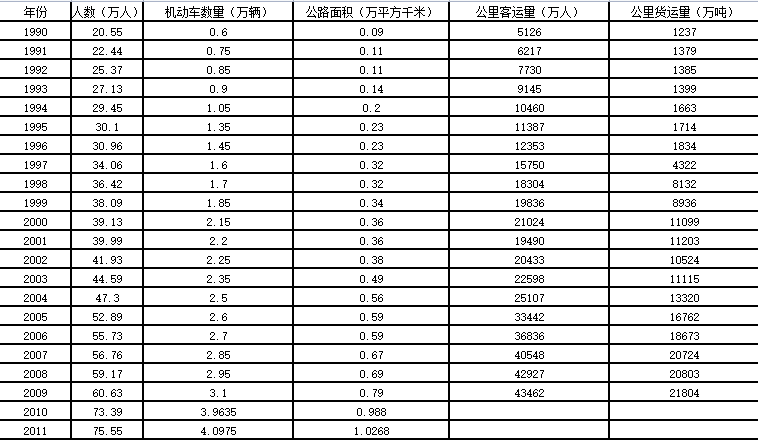#1．数据获取
import pandas as pd
x = data.iloc[:20,:4]
y = data.iloc[:20,4:]
#2．导入线性回归模块,简称为LR
from sklearn.linear_model import LinearRegression as LR
lr = LR()             #创建线性回归模型类
lr.fit(x,y)         #拟合
slr=lr.score(x,y)   #判定系数 R^2
c_x=lr.coef_         #x对应的回归系数
c_b=lr.intercept_   #回归系数常数项
#3．预测
x1 = data.iloc[20:,:4]
r1=lr.predict(x1)    #采用自带函数预测
#print('x回归系数为：',c_x)
#print('回归系数常数项为：',c_b)
#print('判定系数为：',slr)
#print('样本预测值为：',r1)

n=list(data["公里客运量（万人）"])
n.extend(r1[:,0])
num=pd.DataFrame(n).dropna()

g=list(data["公里货运量（万吨）"])
g.extend(r1[:,1])
gravity=pd.DataFrame(g).dropna()

import pandas as pd
import matplotlib.pyplot as plt  #导入绘图库中的pyplot模块,并且简称为plt

#构造绘图所需的横轴数据列和纵轴数据列
#在figure界面上绘制线性图
plt.rcParams['font.sans-serif'] = 'SimHei'     #设置字体为SimHei

plt.figure(1)
plt.plot(data["年份"],num,'r*--')  #红色“*”号连续图,
plt.xlabel('日期')
plt.ylabel('公里客运量（万人')
plt.title('公里客运量（万人)走势图')
plt.xticks(data["年份"],rotation = 45)
plt.savefig('myfigure1')

plt.figure(2)
plt.plot(data["年份"],gravity,'b*--')  #红色“*”号连续图,
plt.xlabel('日期')
plt.ylabel('公里货运量（万吨）')
plt.title('公里货运量（万吨）走势图')
plt.xticks(data["年份"],rotation = 45)
plt.savefig('myfigure2')

from sklearn.neural_network import MLPRegressor
clf = MLPRegressor(solver='lbfgs', alpha=1e-5,hidden_layer_sizes=8, random_state=1)
clf.fit(x, y);
rv=clf.score(x,y)
r2=clf.predict(x1)
print('样本预测值为：',r2)

n2=list(data["公里客运量（万人）"])
n2.extend(r2[:,0])
num2=pd.DataFrame(n2).dropna()

g2=list(data["公里货运量（万吨）"])
g2.extend(r2[:,1])
gravity2=pd.DataFrame(g2).dropna()

结果显示：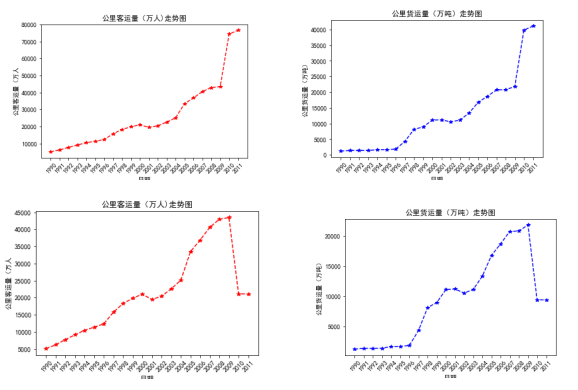展开全文神经网络 线性回归 机器学习
• 参考链接中的文章，错误，我给更正了。 并且原文中是需要数据集文件的，我直接给替换成了一个数组，采用直接赋值的方式。 # -*- coding: utf-8 -*- import numpy as np import matplotlib.pyplot as plt class ...

参考链接中的文章，有错误，我给更正了。

并且原文中是需要数据集文件的，我直接给替换成了一个数组，采用直接赋值的方式。

# -*- coding: utf-8 -*-
import numpy as np
import matplotlib.pyplot as plt

def __init__(self, data_file):
self.train_file_name = data_file
self.num_train = 0
self.XTrain = None
self.YTrain = None

# self.XTrain = data["data"]
# self.YTrain = data["label"]
self.XTrain = np.array([0.95, 3, 4, 5.07, 6.03, 8.21, 8.85, 12.02, 15], dtype=float)
self.YTrain = np.array([5.1, 8.7, 11.5, 13, 15.3, 18, 21, 26.87, 32.5], dtype=float)
self.num_train = self.XTrain.shape
#end if

# get batch training data
def GetSingleTrainSample(self, iteration):
x = self.XTrain[iteration]
y = self.YTrain[iteration]
return x, y

def GetWholeTrainSamples(self):
return self.XTrain, self.YTrain

class NeuralNet(object):

def __init__(self, eta):
self.eta = eta
self.w = 0
self.b = 0

def __forward(self, x):
z = x * self.w + self.b
return z

def __backward(self, x,y,z):
dz = z - y                  # 原错误为：dz = x * (z - y)
db = dz
dw = dz
return dw, db

def __update(self, dw, db):
self.w = self.w - self.eta * dw
self.b = self.b - self.eta * db

# get x and y value for one sample
# get z from x,y
z = self.__forward(x)
# calculate gradient of w and b
dw, db = self.__backward(x, y, z)
# update w,b
self.__update(dw, db)
# end for

def inference(self, x):
return self.__forward(x)

if __name__ == '__main__':
# create net
eta = 0.1
net = NeuralNet(eta)
net.train(sdr)
# result
print("w=%f,b=%f" %(net.w, net.b))
# 绘图部分
trainX,trainY = sdr.GetWholeTrainSamples()
fig = plt.figure()
# 绘制散点图
ax.scatter(trainX,trainY)
# 绘制线性回归
x = np.arange(0, 15, 0.01)
f = np.vectorize(net.inference, excluded=['x'])
plt.plot(x,f(x),color='red')
# 显示图表
plt.show()Ref：

1. 通过神经网络实现线性回归的拟合
展开全文神经网络 线性回归 python
• 定义网络 class LinearRegressionModel(nn.Module): def __init__(self, input_shape, output_shape): super(LinearRegressionModel, self).__init__() # 第一步必须这么写 self.linear = nn.Linear(input_shape,...

定义网络

class LinearRegressionModel(nn.Module):
def __init__(self, input_shape, output_shape):
super(LinearRegressionModel, self).__init__() # 第一步必须这么写
self.linear = nn.Linear(input_shape, output_shape)

def forward(self, x): # 反向传播
out = self.linear(x)
return out

全部代码

import torch
import torch.nn as nn

class LinearRegressionModel(nn.Module):
def __init__(self, input_shape, output_shape):
super(LinearRegressionModel, self).__init__()
self.linear = nn.Linear(input_shape, output_shape)

def forward(self, x):
out = self.linear(x)
return out

if __name__ == '__main__':
x_train = torch.randn(100, 4)  # 生成100个4维的随机数，作为训练集的 X
y_train = torch.randn(100, 1)  # 作为训练集的label

# 指定参数与损失函数
model = LinearRegressionModel(x_train.shape, 1)  # 对应__init__方法的input_shape:输入的维度；output_shape:输出的维度
epochs = 1000  # 迭代1000次
learning_rate = 0.01  # 学习率
optimizer = torch.optim.SGD(model.parameters(), lr=learning_rate)  # 优化函数
criterion = nn.MSELoss()  # Loss使用MSE值，目标是使MSE最小

for epoch in range(epochs):
epoch += 1
# 梯度要清零每一次迭代
# 前向传播
outputs = model(x_train)
# 计算损失
loss = criterion(outputs, y_train)
# 返向传播
loss.backward()
# 更新权重参数
optimizer.step()
# 每隔50次打印一次
if epoch % 50 == 0:
print('epoch {}, loss {}'.format(epoch, loss.item()))
# 预测
predicted = model(torch.randn(100, 4)).data.numpy()
print(predicted)

展开全文pytorch
• 背景：一直想要梳理一下自己对广义线性模型的认识及思考，所有就了这篇随笔。 前提： ...一、线性回归 该算法主要拟合一个线性超平面，用于回归任务（预测值为连续值）。举个例子，房价预测任务，如...

背景：一直想要梳理一下自己对广义线性模型的认识及思考，所有就有了这篇随笔。

前提

1、首先明确，介绍模型会按照模型的三要素来展开，即模型（模型的参数空间），策略（如何选择最优模型，一般指代价函数/损失函数），算法（模型学习参数的方法，包括最优化方法等）

2、因为介绍的模型都是线性模型及其演化，有个概念必须要介绍

超平面：N维空间的N-1维子空间，比如当N=2时，超平面就是二维空间中的一条线

一、线性回归

该算法主要拟合一个线性超平面，用于回归任务（预测值为连续值）。举个例子，房价预测任务，如果只考虑房屋面积，则训练出的超平面及为二维空间关于面积和房价的直线。

模型：

若样本用d维特征来表示，则有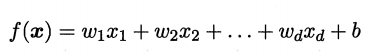使用向量的形式表示为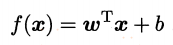则模型的参数空间为w及b

策略：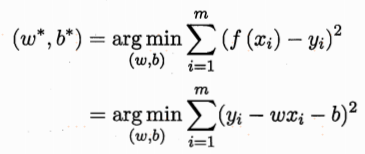最小化均方误差

算法：

这里有两种方法，

一种方法是算出参数的表达式，直接求解（该方法一般不使用，原因随着特征或者样本数量增长，计算的时间复杂度指数级增加），如下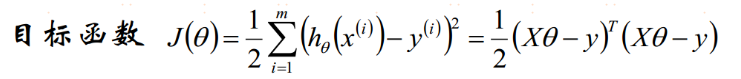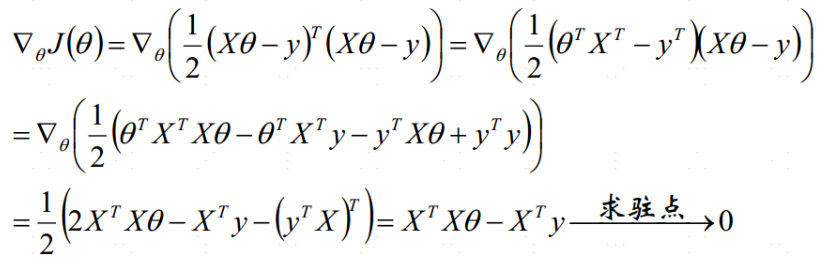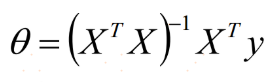另一种方法是最优化理论中的迭代方法，最常用的是梯度下降法，参数通过加上负梯度进行更新，

求梯度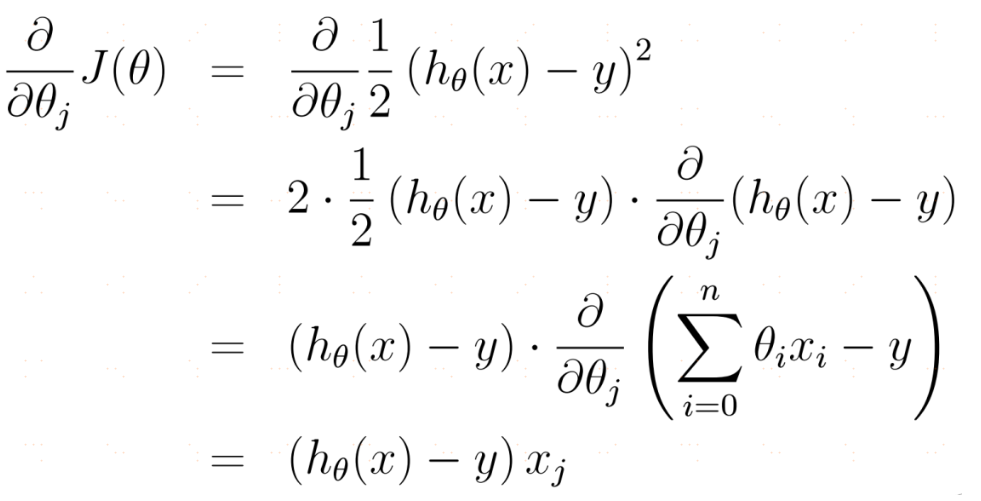使用梯度对参数进行更新（这里使用的是批梯度下降算法）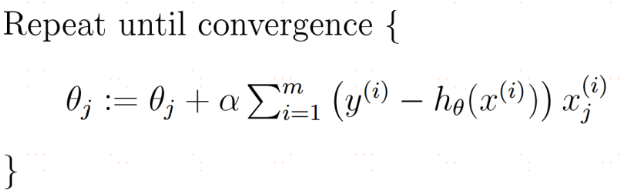问题：当目标变量与特征之间为非线性关系，我们又该怎样去拟合呢

二、多项式回归

思路如下图所示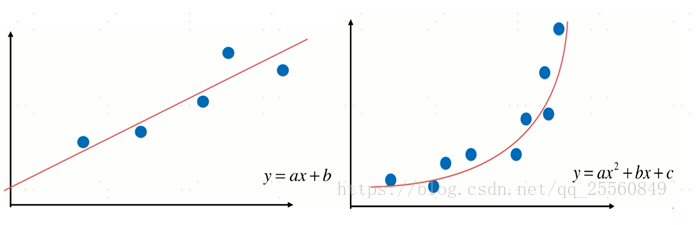模型整体和线性回归相似，所以这里不展开讨论

问题：线性回归可以解决回归问题，那针对分类问题，我们应该怎样用线性模型去解决呢？

三、感知机

线性回归在N维空间中找到了线性超平面去拟合数据，同时，该线性超平面也把特征空间划分成了两份，据此，我们可以思考，这个超平面可以拿来进行分类吗？当然可以，感知机就是这样做的。

模型：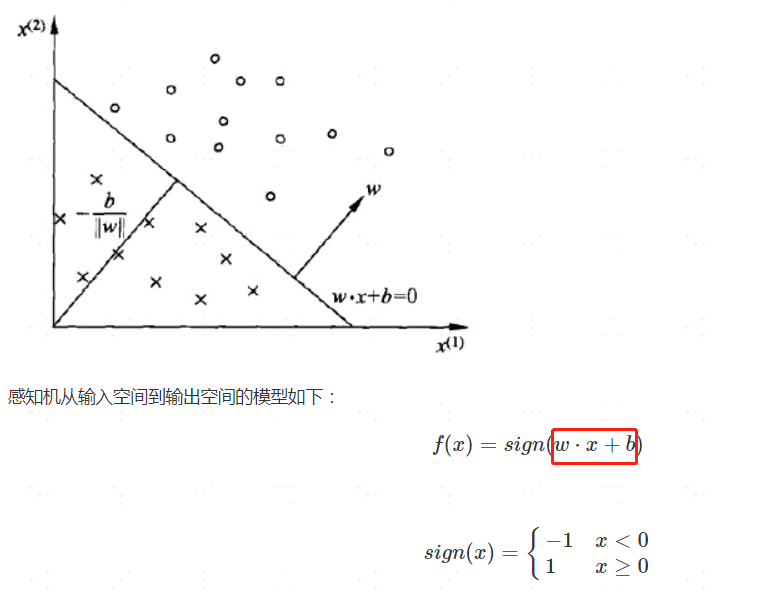我们可以看到，感知机就是使用sign函数对线性回归结果映射到了{-1,1}

策略：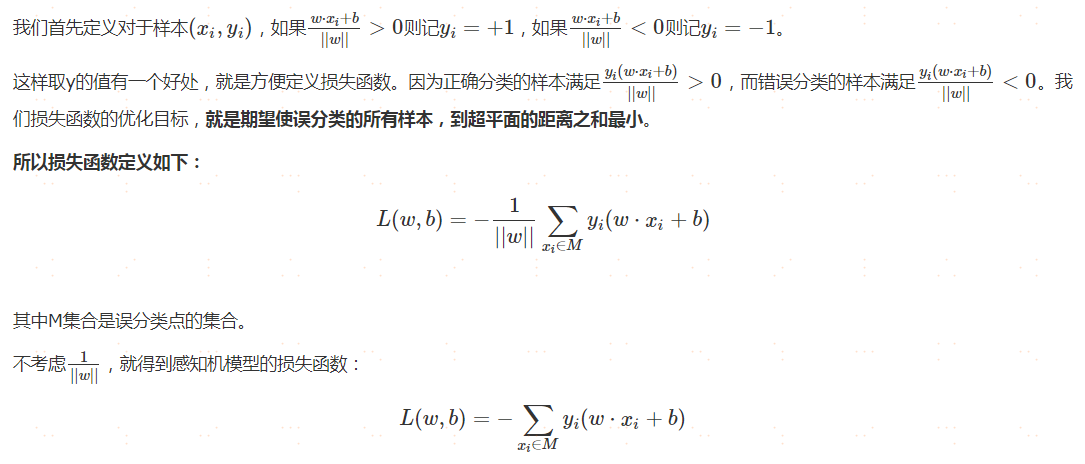算法：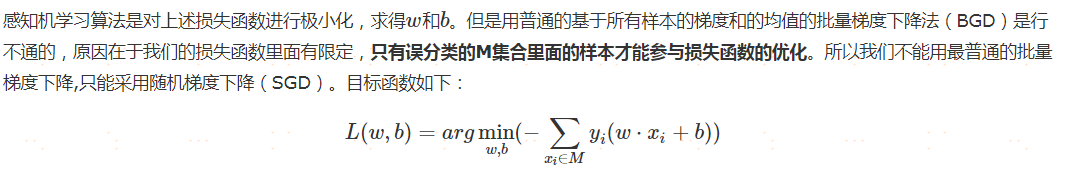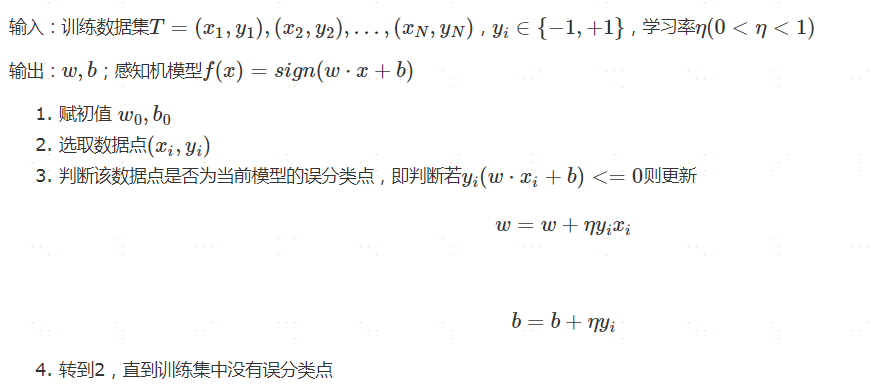问题：感知机可以用于分类，且能够输出预测样本对应的类别，但清楚感知机原理的我们知道，虽然有可能N个样本都被预测为A类，但实际他们属于A类的可能性是不同的，具体表现在有的样本点在特征空间中距离分类超平面比较近，有的则比较远；鉴于此，我们能不能输出某样本属于A类别的概率呢？

四、逻辑回归（logistics regression）

模型：

感知机使用线性回归模型作为分类超平面，将线性回归结果映射到{-1,1}的离散集合上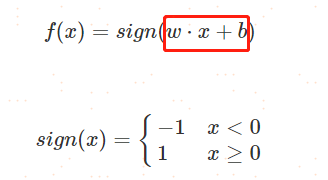其中红框的值域为负无穷到正无穷，那我们能不能使用一个函数将其映射到[0,1]呢。逻辑回归便是选择了sigmoid函数进行映射，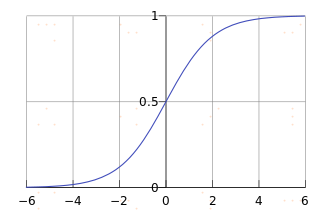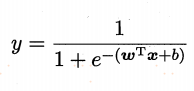该函数既可以实现区间映射，又具有单调可微的特性（方便使用迭代的最优化方法计算模型参数）

另外，对于“逻辑回归”的名字，很多人觉得这是个“假名”，“误导人”，认为它明明是分类算法却叫做“回归”

其实，他还真的和名字所述，是对“对数”进行了“回归”

上一个公式可以变化为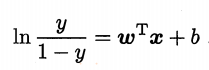定义y/1-y为事件发生的“几率”，则逻辑回归的是指是对几率的对数进行回归拟合。

策略：

采用极大似然估计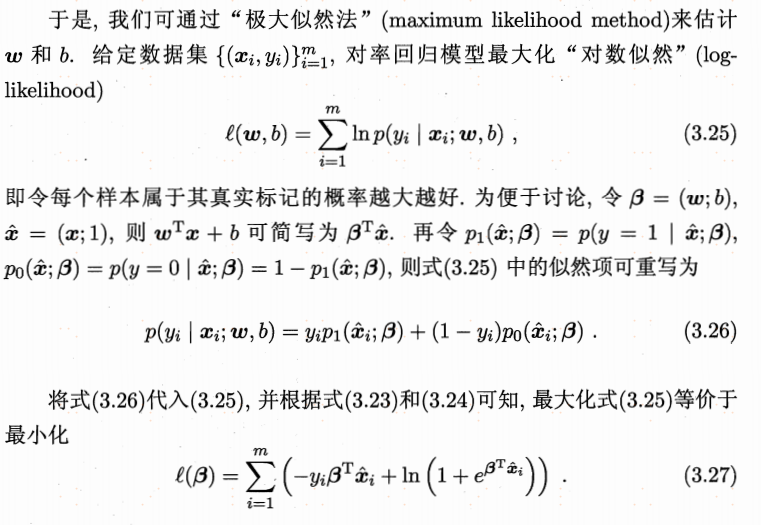算法：

由于目标函数连续可导，根据凸优化理论，可以通过一阶法（梯度下降等）或二阶法（牛顿法）等进行参数迭代计算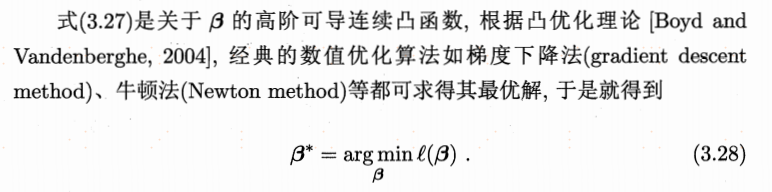问题：对于分类任务，感知机和逻辑回归都只能处理线性可分的任务，那如何才能处理线性不可分的任务呢？而且按照之前感知机迭代求解分类超平面的方式，我们只是在众多的符合条件的分类超平面中选择了一个，那能否选择一个相对更好一些的分类超平面呢？

五、支持向量机（SVM）

支持向量机通过以下思路解决了上述问题：

（1）针对分类超平面的选择问题，SVM采用最大间隔的方法，使超平面距离两侧不同类别的点尽可能地远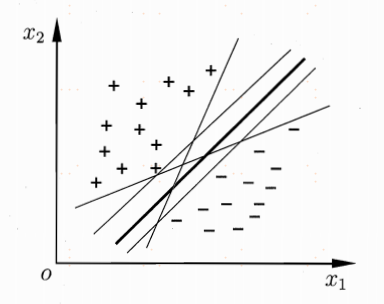（2）针对在当前维度的特征空间下线性不可分的问题，我们对其进行维度扩张，在更高的维度对其进行线性划分。在当前维度线性不可分不代表在更高维度线性不可分。举个例子，演员拍摄吻戏经常采用错位的方式，在镜头所能看到的二维平面中，找不到一条直线将他们的嘴分割开来，但考虑三维空间，在一个现场工作人员看来，有大量的可选平面将他们两人的嘴分开。再比如下图中的异或问题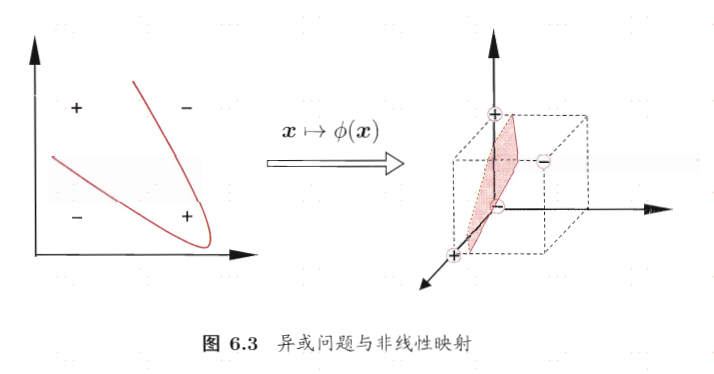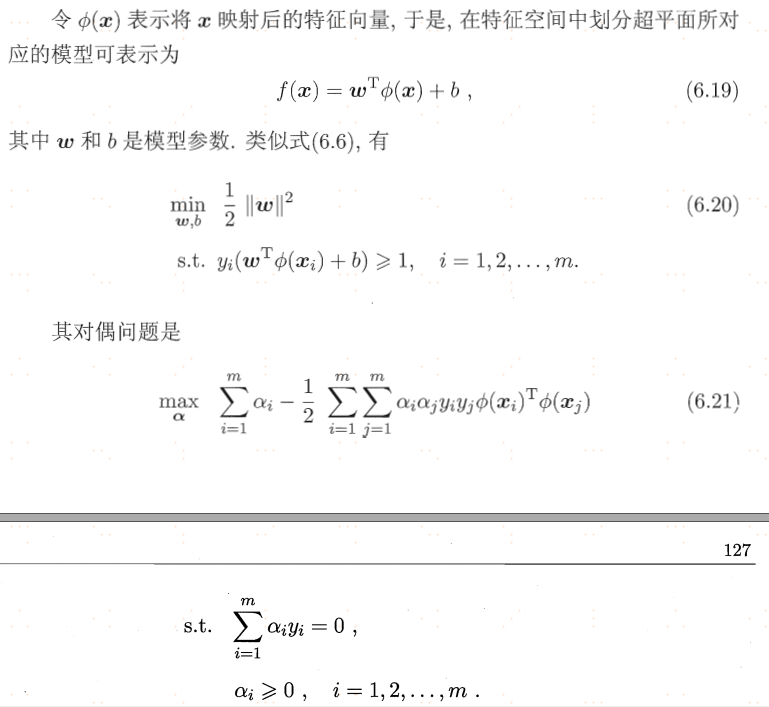接下来又有另一个问题，我们已经用高维向量计算表示出了最大分类间隔，那怎样去表示具体的高维向量呢，考虑到维度可能会很高，计算复杂度可能会很大，我们可以采用核函数来进行等价计算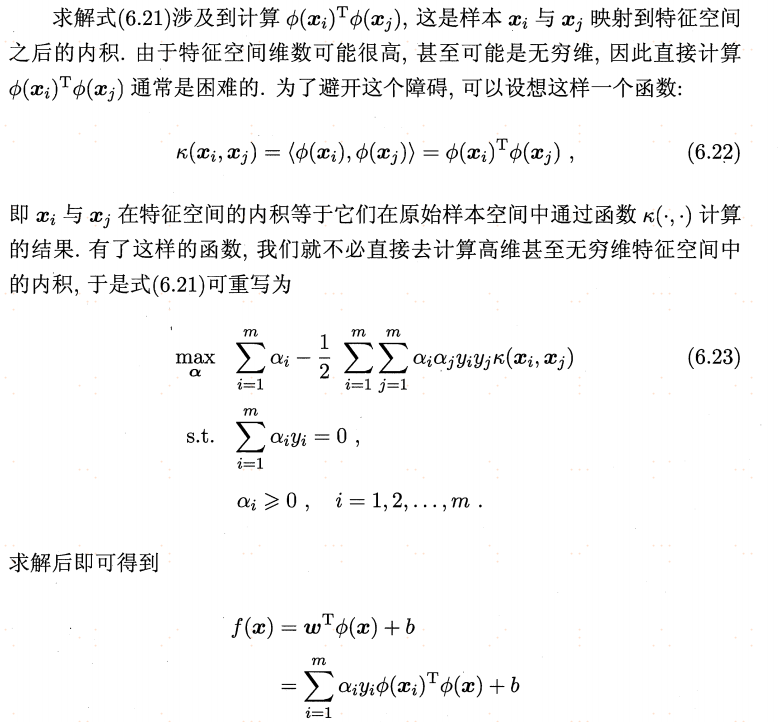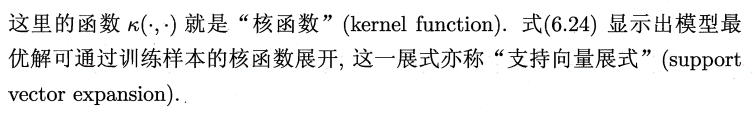支持向量机的核心就是以上两点，更多细节可以看一下其他的博客或书籍，篇幅原因不再展开

问题：除了SVM之外，还有其他方法可以解决线性不可分问题吗？

六、神经网络

神经网络通过特征之间的组合（多层），不仅能够解决线性不可分问题，还能够保留数据的时空结构（有位置关系的图像数据和有时间先后关系的自然语言），再该类数据集上表现出强大的应用能力。

总之，当前kaggle上表现最好的两类算法，梯度提升算法更加适合于表格类型结构的数据，需要进行特征工程，对少量样本也能学习到较好的模型；神经网络更加适合有时空结构的数据，不需要复杂的特征工程，是端对端的学习，但需要大量的训练数据作为支撑。

个人认为神经网络的强悍之处在于对时空数据的保留与学习能力+无处不在的特征组合。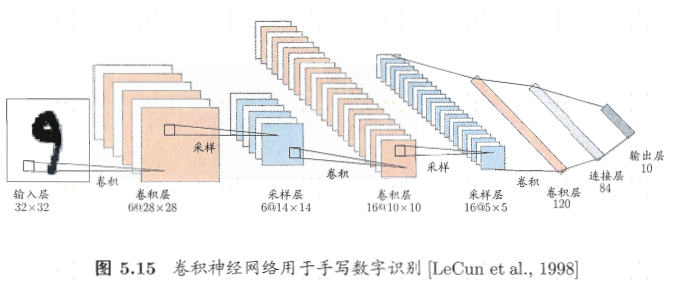神经网络具体内容略多，不展开啦，本文重点梳理思路。

参考：

https://www.cnblogs.com/huangyc/p/9706575.html
https://blog.csdn.net/qq_36523839/article/details/82924804

李航-统计学习方法

周志华-机器学习

展开全文• 1.神经网络实现非线性回归 import torch import matplotlib.pyplot as plt import os os.environ["KMP_DUPLICATE_LIB_OK"] = "TRUE" x = torch.unsqueeze(torch.linspace(-3, 5, 421), dim=1) y = 0.3 * x.pow(2) +...神经网络 回归 python
• 用pytorch实现神经网络线性回归 import torch import matplotlib.pyplot as plt from torch import nn, optim from time import perf_counter x = torch.unsqueeze(torch.linspace(-3, 3, 10000), dim=1) y = x + ...机器学习 深度学习
• 0.导入相应的API import torch # 导入pytorch from torch import nn # 导入nn.module from torch import optim # 导入优化器类api optimizer import numpy as np # 导入numpy 数据计算 from matplotlib import ...神经网络 pytorch 深度学习
• python线性回归

2020-12-30 12:23:22
对于多元线性回归，我们：或者为了简化，干脆将b视为k0·x0,，其中k0为1，于是我们就：２.损失函数3.误差衡量MSE,RMSE,MAE越接近于0越，R方越接近于1越。MSE平均平方误差(mean squared error)RMSE，是MSE的...
• 什么线性分类器 寻找一个函数 把D维空间的输入映射到K维空间（分为K列） 输入是D维列向量，输出是K*1维，那么W一定是K*D维 Wx+b的物理意义是什么 wx是列向量的内积->就是在算两个列向量的相关度->是在...
• 多元线性回归算法预测房价一、理论学习（一）背景（二）线性回归检验二、数据清洗（一）数值数据处理（二）非数值型数据转换三、Excel多元线性回归四、多元线性回归模型预测房价（一)基础包与数据导入（二）变量探索...算法 线性回归 回归
• 什么线性回归？简单举个例子，给定一个直线方程 y=kx+by=kx+by=kx+b 和位于该直线上的两点(x1,y1)(x_1,y_1)(x1​,y1​)、(x2,y2)(x_2,y_2)(x2​,y2​)。问x=x3x=x_3x=x3​时，y=y3=?y=y_3=?y=y3​=? 根据中学知识...神经网络 pytorch 深度学习 tensorflow 机器学习
• 一、一元线性回归一元线性回归是回归分析中最简单的一种形式，主要考察单独1个自变量对因变量的影响。其模型形如: y=a+ βx+ε一元线性回归分析的基本步骤如下：依据变量之间的关系，判断其是否是线性关系。如果是...
• 以上矩阵的基本操作： 接下来是线性回归的计算： imort numpy as np from numpy.linalg import inv from numpy import dot from numpy import mat #计算y=2x x=mat([1,2,3]).reshape([3,1]) y=2*x #再计算theta=(x'...
• 线性回归阶段学习总结

千次阅读 2021-12-11 18:58:40
一、如何理解线性回归模型 简单举个例子：期末总成绩=0.6x 平时成绩+ 0.3 x 期末考试成绩 + 0.1 x 考勤 期末总成绩为目标值，平时成绩、期末考试成绩、考勤是特征值，在目标值和特征值之间建立一个关系，这个关系...线性回归 算法 回归
• 线性回归 import torch import numpy as np import matplotlib.pyplot as plt %matplotlib inline from torch import nn,optim from torch.autograd import Variable import os os.environ["KMP_DUPLICATE_LIB_OK"]=...网络 神经网络 python 深度学习 机器学习
• 线性回归基本可以是LR的简化版（论证思路上），因此本文将主要探讨两个问题：1. 为什么LR可以将权重初始化为0？2. 为什么神经网络不能将权重初始化为0？ 本文参考资料：https://zhuanlan.zhihu.com/p/75879624 为...神经网络 机器学习 算法 LR
• 线性回归输出的是一个真实值，更适用于预测连续的数值预测问题，损失函数可以使用MSE（均方误差），最后的激活函数不要使用sigmod函数 逻辑回归输出的是一个概率值，更适用于离散的分类问题，损失函数使用MSE没有...逻辑回归 深度学习 机器学习 神经网络 线性回归
• 1. 机器学习眼中的线性回归左图是原始建模数据，目的是用来寻找玩偶数量和成本的某种关系。右图将数据可视化得到散点图。第一步 进行场景确定第二步 定义损失函数建模的目的：模型预测值与真实值之间的差距越小越...
• tensorflow神经网络 # import tensorflow as tf import tensorflow.compat.v1 as tf tf.disable_v2_behavior() import matplotlib.pyplot as plt import numpy as np a = 2 # 权重 b = 1.5 # 偏置 # 设置随机...神经网络
• 深度学习 —— 从 *print（‘hello world’）* 到 *sending paper*前言主要内容代码 前言 ...学透本文的 线性回归 神经网络，以后就可以举一反三。 为了完全弄透，手写了一份代码，记录如下。 主要内容深度学习 python 机器学习 神经网络
• 线性回归中的L1与L2正则化

千次阅读 2021-02-26 08:51:16
一种方法可以对损失函数的过拟合进行调整，那就是惩罚。通过惩罚或“正则化”损失函数中的大系数，我们使一些(或所有)系数变小，从而使模型对数据中的噪声不敏感。 在回归中使用的两种流行的正则化形式是L1又名...机器学习 线性回归 Lasso回归 岭回归 正则化
• 线性回归总结

2020-12-26 01:11:34
文章目录线性回归线性回归简介线性回归应用场景什么线性回归定义与公式线性回归的特征与目标的关系分析线性回归api初步使用线性回归API举例数学:求导常见函数的导数导数的四则运算练习3.2 y=ln(sinx), 求dy/dx矩阵...python 算法 机器学习
• 导读：本文将介绍什么回归问题、解决回归问题的基本思路步骤和用机器学习模型解决回归问题的基本原理，以及如何用线性模型解决回归问题。作者：莫凡来源：大数据DT（ID：hzdashuju）00...算法 大数据 编程语言 机器学习 人工智能
• 在详细了解线性回归的所有知识点之前，我们先来了解一下线性回归的重要性。 理论层面的重要性 Linear Regression：是回归问题的基础 Logistic Regression：是分类问题的基础 可扩展性：使用基函数来解决非线性...机器学习 人工智能 线性回归
• 简单线性回归matlab实现

千次阅读 2021-02-01 10:56:16
为考试方便，用matlab写了个简单线性回归的整套过程，包括所有参数检验和估计。意义不是很大，因为这些用SPSS等统计软件可以很快得出，但统计软件毕竟是黑箱，对原理感兴趣或熟悉的可以看看代码(其实就是写着玩的，...
• 基于tensorflow keras的线性回归： import keras #导入keras import numpy as np #导入numpy用于提供多维数组模型 import matplotlib.pyplot as plt #导入画图的工具包 from keras.models import Sequential #...tensorflow keras 线性回归
• 线性回归中的"线性" 线性回归中的线性和我们通常理解的线性有着本质的区别，例如： X为自变量 函数的次数指的是自变量的最高次项，线性函数表示自变量的最高次项为1；在高中和大学的学习中，我们往往要求解...线性 梯度下降 线性回归 逻辑回归 最小二乘
• 译者序前言第1章　导引11.1　回归与建模11.2　数据收集41.3　回归的用途71.4　计算机的角色7第2章　简单线性回归92.1　简单线性回归模型92.2　回归参数的最小二乘估计92.2.1　β0与β1的估计92.2.2　最小二乘估计量......